# Electronic Reporting in Depth, Format Mapping part 3 Transformations1 Blog Post 13 Header Image

This blog post will explain the concept of the format mapping in Electronic Reporting. After this blog post you should have a general idea on how to create a format mapping and the designer of the format configuration in Electronic Reporting.

Contents

This post assumes that you are familiar with the basics of Electronic Reporting and that you have a good understanding of data models and model mappings. Due to the size and complexity of a format mapping this topic will be split up into multiple posts.

In this post we will focus on the transformations and how you can use these in your configurations.

## Transformations General

Electronic Reporting facilitates the import and export of files to or from data sources. However, it can happen that there are requirements to the exported files, like specific characters that are not allowed. For this purpose, transformations can be used.

A transformation is a formula which you can define and can bind to your format nodes. In this formula you can set up the transformation rules you wish to apply to the nodes bound data. Because you predefine the transformations, you only have to set this up once and you can bind these multiple times to output nodes.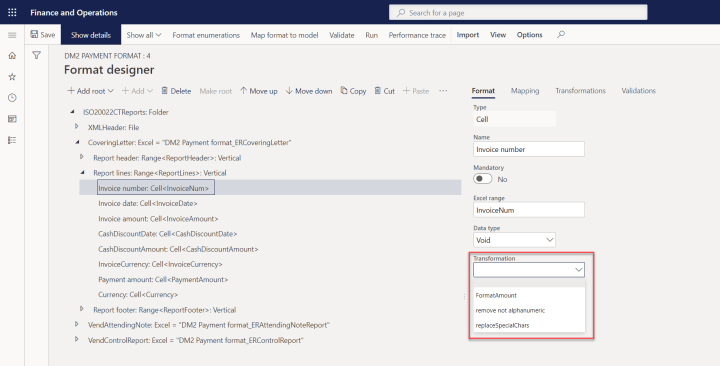Apply Transformation to Node

Transformation setup can be found in the format mapping area.Transformations

In the transformation menu you can see the transformations you have created, and you see three fairly straightforward menu items, New, Edit and Delete. When creating a new transformation or editing an existing transformation the transformation formula menu will appear.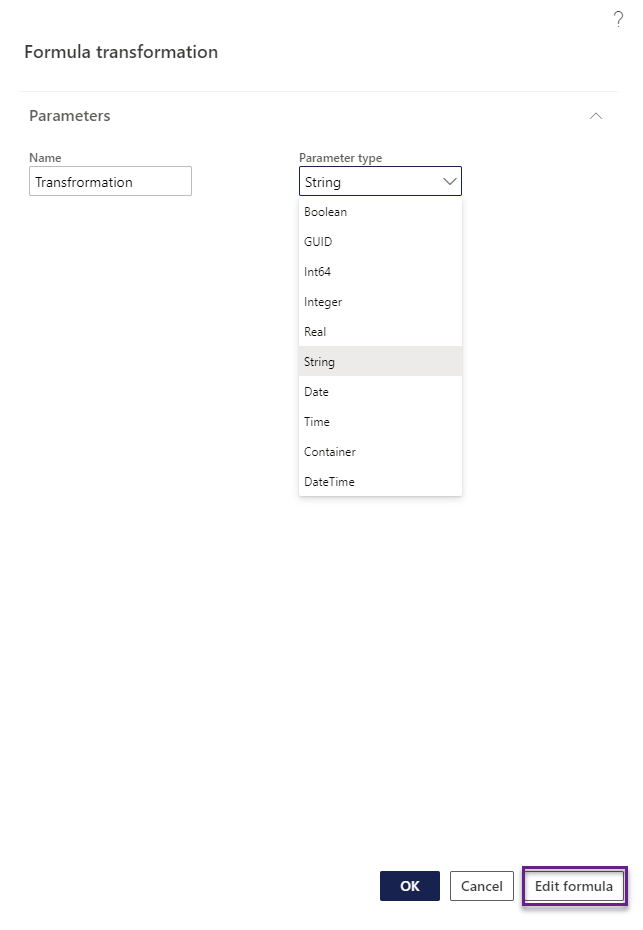New Transformation

As you can see the transformation relies on an input parameter type, this data type should match the bound value on the node you are creating or using the transformation for. The input parameter will be the only data source you can use for transformations.

After defining the name and parameter type you must click on Edit Formula to edit the transformation formula. The type of formulas you will use here are formatting formulas, we will cover the most common ones in the next topics.

## Translate formula

The translate formula is a string-based formula, so the parameter type has to be string. The translate formula has the following structure:

``TRANSLATE(input,pattern,replacement)``

This breaks down to:

• Input, put the input parameter data source here
• Pattern, this is the input string that the formula will search for. Each character put in the pattern is a character which will be replaced by the replacement character. This field is case sensitive
• Replacement, this is the replacement string that the pattern characters will be replaced by. This field is case sensitive. The connection between the pattern characters and the replacement characters is based on the position of the character. The first character in the pattern will be replaced by the first character in the replacement, the second character in the pattern will be replaced by the second character in the replacement, and so on…

The formula translate(parameter,”abcd”,”1234″) visualized looks like this:Translate formula

This formula will replace all a’s with 1, all b’s with 2, all c’s with 3 and all d’s with 4. This results in:Translate result 1

Due to the setup of this formula, it is mandatory to provide a replacement value for each pattern value. Should we change the formula to translate(parameter,”abcd”,”123″) we will see that the d’s will no longer get translated:Translate result 2

A common use for this formula is to remove all special characters and replace them by a normal character (so replace À with A). This formula is taken from the ISO Credit transfer configuration provided by Microsoft, as a SEPA file can not contain these special characters:

``TRANSLATE(parameter, "¿ÀÁÂÃÄÅÆÇÈÉÊËÌÍÎÏÐÑÒÓÔÕÖØÙÚÛÜÝßàáâãäåæçèéêëìíîïðñòóôõöøùúûüýþÿĀāĂăĄąĆćĊċČčĎďĐđĒēĖėĘęĚěĜĝĞğĢģĪīĮįİıĲĳĶķĹĺĻļĽľŁłŃńŅņŇňŐőŒœŔŕŘřŚśŞşŠšŢţŤťŪūŮůŰűŲųŸŹźŻżŽž€&;[\]_{|}~`", "?AAAAAAACEEEEIIIIDNOOOOOOUUUUYsaaaaaaaceeeeiiiidnoooooouuuuytyAaAaAaCcCcCcDdDdEeEeEeEeGgGgGgIiIiIiIiKkLlLlLlLlNnNnNnOoOoRrRrSsSsSsTtTtUuUuUuUuYZzZzZzE+,(/)-(/)-''")``

Besides this you can use the following formula to replace all tabs and enters in your output file with spaces, since this can result in unwanted formatting sometimes as well:

``Translate(parameter,char(9)&char(10)&char(13),"   ")``

You will typically use this formula for these use cases, to replace the input character while not losing any usability on your file (as removing all A’s from the file will result in an unusable or incorrect file). However, due to the setup of the translate formula, you can not use this formula to remove characters you do not want, you always have to provide a replacement value.

## Replace formula

The replace formula is a string-based formula as well. This formula is much like the translate formula with only a few specific differences, the replace formula has the following structure:

``REPLACE(input,pattern,replacement,is_regular_expression)``

This breaks down to:

• Input, put the input parameter data source here
• Pattern, this is the input string that the formula will search for. Where the translate formula requires you to input each character you would like to use, the replace formula can work with ranges.
• [  ], everything between this is regarded as a range. This will change lookup to a contains method as well. This will be explained with examples further on.
• -, can be used to create a range. So the range abcd can be written down as [abcd], but you could use [a-d] as well
• ^, this character is an important character. Normally the replace function is used like the search and replace function, so all values in the pattern string will be replaced. The ^ symbol changes this behavior, this symbol makes the replace function act as does not contain and replace, so all values except for the pattern string will be replaced.
• Replacement, this is the replacement string that the pattern characters will be replaced by. Where the translate formula translates each character in the input with the replacement character, the replace formula will replace each character found in the pattern with the characters in the replacement. So most commonly your replacement character will either be “” which removes the characters found in the pattern or ” ” which replaces the characters found in the pattern with a space. So unlike the translate function, the pattern and replacement do not have to be of the same length.
• Regular expression, can be either true or false and this setting affects the behavior of the pattern
• True, this will make the formula look for a contain match which means that you can work with ranges.
• False, this will make the formula look for an exact match with the pattern input. This will disable the range input on the pattern as well, as the [] or ^ will be detected as characters.

As examples different variations of the REPLACE(parameter, “abcd”, “1”, true) will be used. As input string abcdefgh and hgfedcba will be used.

Formula REPLACE(parameter, “abcd”, “1”, true), will search for a contain match with abcd and replace the matched string with 1, results: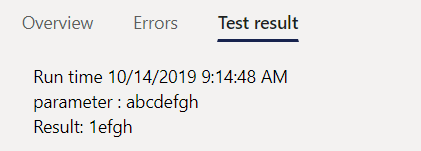Replace result 1a

Formula REPLACE(parameter, “abcd”, “1”, false), will search for an exact match with abcd and replace the matched string with 1, results: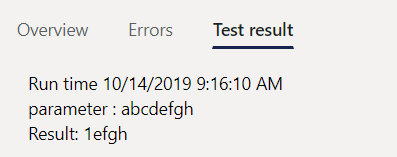Replace result 2a

Formula REPLACE(parameter, “^abcd”, “1”, false), will search for an exact match with ^abcd (the ^ is seen as a character due to the false parameter on the regular expression) and replace the matched string with 1, results: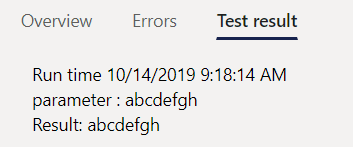Replace result 3a

Formula REPLACE(parameter, “^abcd”, “1”, true), will search for an contain match with ^abcd (the ^ is ignored as a character due to no [] making the match fail, unlike 3c) and replace the matched string with 1, results: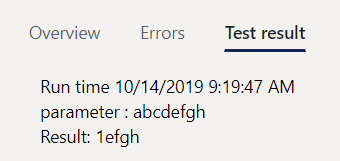Replace result 4a

Formula REPLACE(parameter, “[abcd]”, “1”, true), will search for an exact match with abcd per character and replace the characters string with 1, notice that the reverse string gets changed now as well, results: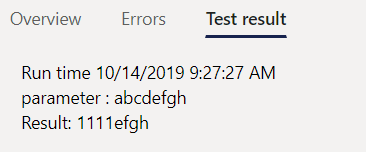Replace result 5a

Formula REPLACE(parameter, “[^abcd]”, “1”, true), will search for any character not being abcd per character and replace these characters string with 1, results: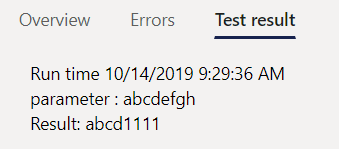Replace result 6a

Formula REPLACE(parameter, “[^abcd]”, “1”, false), will search for the string [^abcd] and replace this string with 1, results: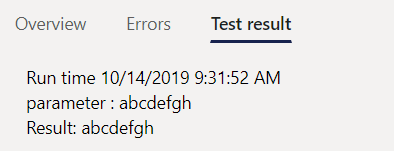Replace result 7a

Formula REPLACE(parameter, “[a-d]”, “1”, true), will search for an exact match with abcd per character and replace the characters string with 1, notice that the reverse string gets changed now as well, results: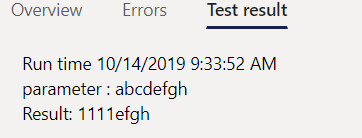Replace result 8a

Formula REPLACE(parameter, “[^a-d]”, “1”, true), will search for any character not being abcd per character and replace these characters string with 1, results:Replace result 9a

As you can see the results for [abcd] and [a-d] are equal.

Typical uses for the replace formula is to validate the input characters, the formula below will remove all characters except for a to z (notice that it is case sensitive) and 0 to 9:

``REPLACE(parameter, "[^a-zA-Z0-9]", "", true)``

When looking at the ISO credit transfer transformations you will find the formula below:

``REPLACE(TRANSLATE(parameter, "¿ÀÁÂÃÄÅÆÇÈÉÊËÌÍÎÏÐÑÒÓÔÕÖØÙÚÛÜÝßàáâãäåæçèéêëìíîïðñòóôõöøùúûüýþÿĀāĂăĄąĆćĊċČčĎďĐđĒēĖėĘęĚěĜĝĞğĢģĪīĮįİıĲĳĶķĹĺĻļĽľŁłŃńŅņŇňŐőŒœŔŕŘřŚśŞşŠšŢţŤťŪūŮůŰűŲųŸŹźŻżŽž€&;[\]_{|}~`", "?AAAAAAACEEEEIIIIDNOOOOOOUUUUYsaaaaaaaceeeeiiiidnoooooouuuuytyAaAaAaCcCcCcDdDdEeEeEeEeGgGgGgIiIiIiIiKkLlLlLlLlNnNnNnOoOoRrRrSsSsSsTtTtUuUuUuUuYZzZzZzE+,(/)-(/)-''"), "[^A-Za-z0-9/\-\?:\(\)\.,''\+ ]", " ", true)``

This formula is a combined formula of the replace and translates. This formula will first replace all special characters with a regular character (so À to A) and after this it will perform an additional validation by removing all characters not alphanumeric and replacing these with a space. In this formula, the replace is basically a catch all, should you have missed some character in the translate formula.

As you can see the replace formula is very useful when you want to remove or replace certain characters.

## Number formatting formula

Sometimes some files require you to have a specific amount of decimals or round of amounts to a certain threshold. This is done by the numberformat formula, which requires a real parameter type.

``NUMBERFORMAT(parameter,format)``

The format can be filled in with:

• , –> thousands separator.
• . –> decimal seperator
• 0 –> number placeholder, if no number is provided a 0 will be displayed
• # –> number placeholder, if no number is provided no number will be displayed

A typical number format is NUMBERFORMAT(parameter, “#,0.##”), this will give you a thousands separator with a 0 display value and 2 decimals if available. So, input 1234567.89 gets formatted to: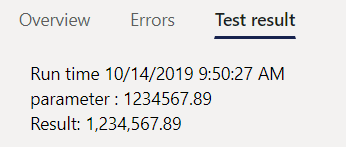Number format result 1

The NUMBERFORMAT(parameter, “#,0,.##”) will round your amounts to millions while providing 2 decimals (after rounding). So, input 1234567.89 gets formatted to: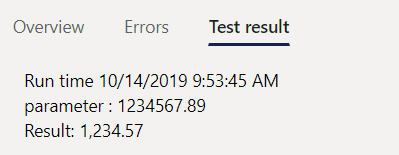Number format result 2

The NUMBERFORMAT(parameter, “#,0,,.##”) will round your amounts to millions while providing 2 decimals (after rounding). So, input 1234567.89 gets formatted to: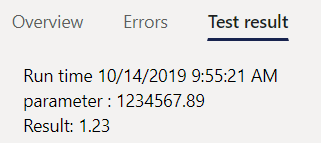Number format result 3

To increase the number of decimals just add more # behind the decimal separator. So the format NUMBERFORMAT(parameter, “#,0.00###”) will add a thousands separator and always display a zero value for the base number and first two decimals. Additional decimals will be shown (up to 3 decimals) if they are present, otherwise they will be hidden:Number format result 4a

## Other formulas

Besides the beforementioned there are other formulas which you can use to your advantage as transformations. Although it is possible to work with date transformations, these have the disadvantage that for some formats the output gets converted to string, which will cause errors if the field expects a date value. So, in general it is not recommended to use transformations for this.

Other formulas include:

• TRIM(input), the trim function allows you to remove leading and trailing spaces in strings, besides this the double spaces between words are removed as well.
• UPPER(input), the upper function will convert all characters in the input string to upper case letters.
• LOWER(input), the lower function will convert all characters in the input string to lower case letters.
• PADLEFT(input,length,padding_chars), the pad left function will add leading characters for the input string up to the specified length. So PADLEFT(parameter,10,”0″) will result for the input into 000000test.

## Conclusion

You now should have a good idea on how to use the transformation functionality for your format configurations in Electronic Reporting. We have discussed commonly used transformation formulas and elaborated on how to use these including some sample formulas. Besides this we have seen a few other formulas you could use to your advantage.